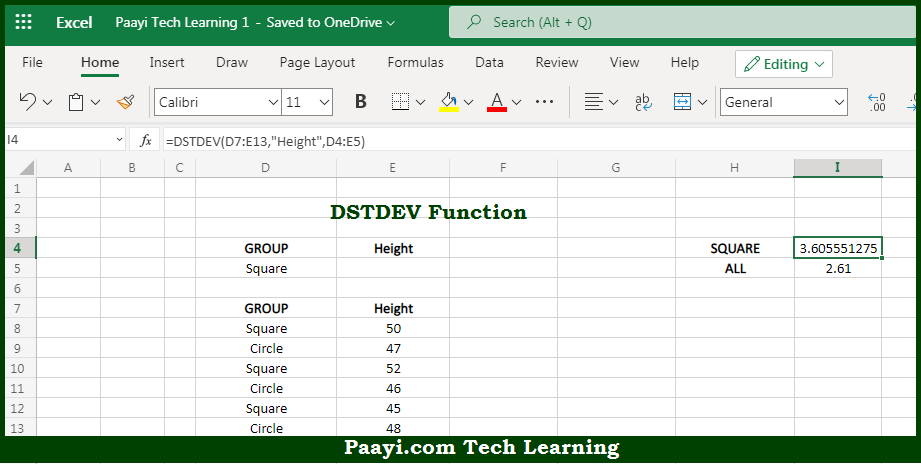# Learn How to Use Microsoft Excel DSTDEV Function

Written by | 0 Comments | 620 Views

In this article, you will learn how to use the Microsoft Excel DSTDEV function and its prime function in Microsoft Excel. You will also get to know the Microsoft Excel DSTDEV function return value and syntax with the help of some examples.

Microsoft Excel DSTDEV Function

The main purpose of the Microsoft Excel DSTDEV function is to get the standard deviation from the matching records in the database. That implies, with the help of the DSTDEV function you can able to return the standard deviation of sample data extracted from records that match the given criteria. You can use the DSTDEVP function in case data represents the entire population. So, with the help of the DSTDEV function, you can able to get the standard deviation from the matching records in the database.

Return Value of DSTDEV Function

The return value will be the calculated standard deviation.

Syntax of DSTDEV Function

=DSTDEV(database, field, criteria)

Where the arguments:

• database: This is the database range including headers.
• field: This is a field name or index to count optional.
• criteria: This is the criteria range including headers.

How to Use Microsoft Excel DSTDEV Function?So we know that Microsoft Excel DSTDEV function you can able to get the standard deviation from the matching records in the database. That implies, with the help of the DSTDEV function you can able to return the standard deviation of sample data extracted from records that match the given criteria. You can use the DSTDEVP function in case data represents the entire population. So, with the help of the DSTDEV function, you can able to get the standard deviation from the matching records in the database.